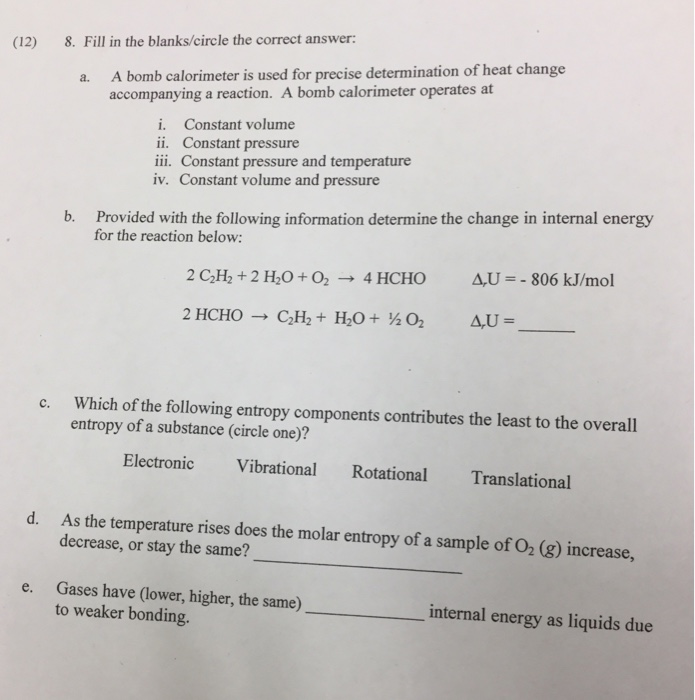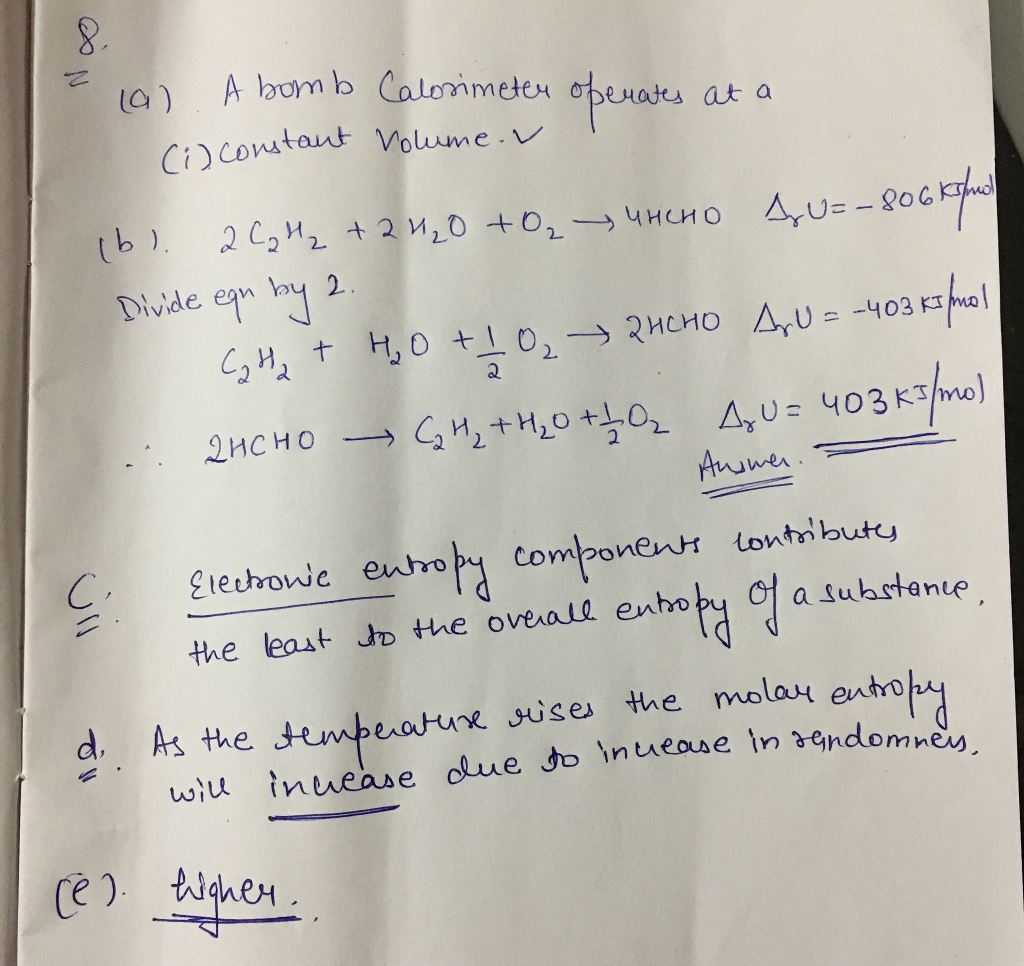# Question & Answer: A. A bomb calorimeter is used for precise determination of heat change accompanying a…..A. A bomb calorimeter is used for precise determination of heat change accompanying a reaction. A bomb calorimeter operates at i. Constant volume ii. Constant pressure iii. Constant pressure and temperature iv. Constant volume and pressure b. Provided with the following information determine the change in internal energy for the reaction below: 2 C_2 H_2 + 2 H_2 O + O_2 rightarrow 4 HCHO delta_r U = -806 kJ/mol @ HCHO rightarrow C_2 H-2 + H_2 O + 1/2 O_2 delta_r U = _______ c. Which of the following entropy components contributes the least to the overall entropy of a substance?. Electronic Vibrational Rotational Translational d. As the temperature rises does the molar entropy of a sample of O_2 (g) increase, decrease, or stay the same? _______ e. Gases have (lower, higher, the same) _________ internal energy as liquids due to weaker bonding.